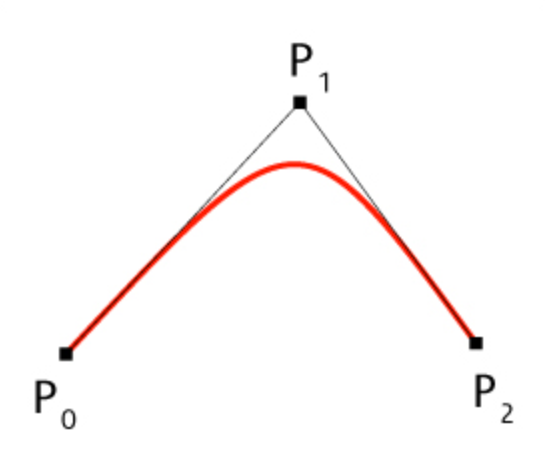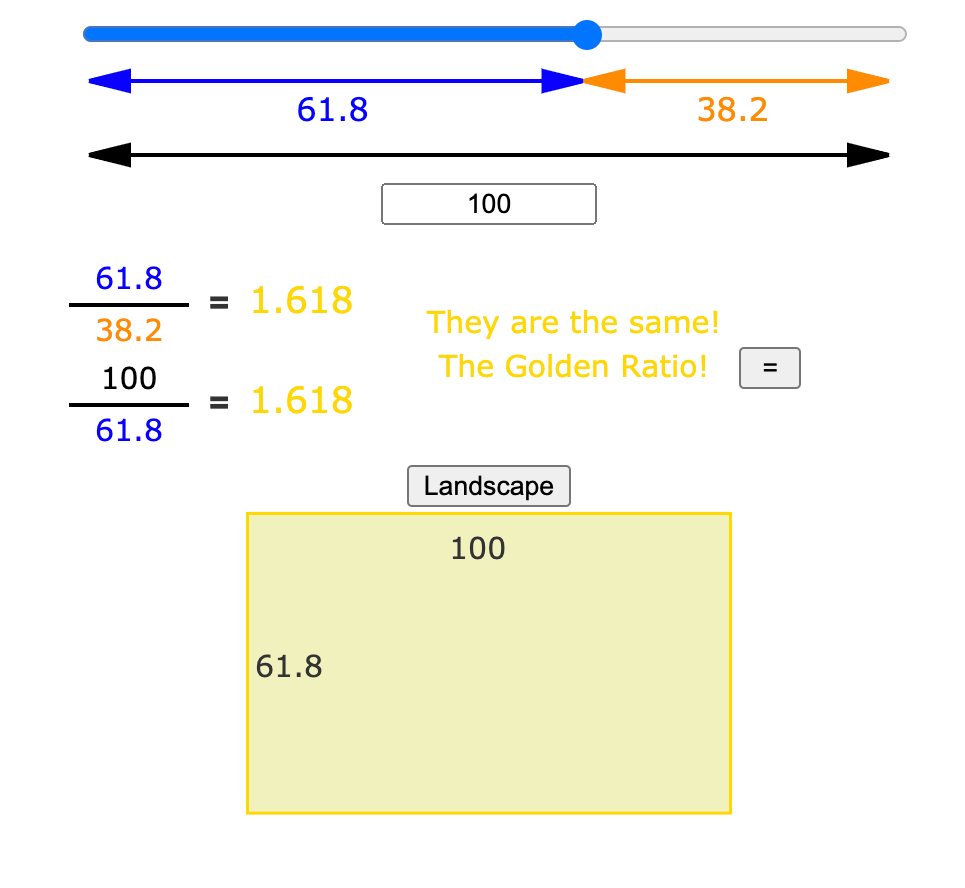Search
•Diana de Avila

# Getting a Little Mathy

Updated: Aug 17, 2021

“Math?”You must be thinking, “Isn’t this blog supposed to be about the book and art?”

Yes, it is. But it’s not about just any kind of art. It’s about digital art. And it’s about the art of an acquired art savant who knows things she has never learned—and that involves the algorithms and math that is apparent in her work. Thus, we want to further acquaint you with the math you’ll find in her work. (Now, who was it who said we’d never use math in our lives?)

Fractal: A fractal is a pattern that repeats forever. Every part of it, regardless of it scale (that is, how zoomed in or out you are) looks similar. Big or small, a fractal is an infinite copy of itself.

The term fractal was coined by Benoît Mandelbrot in 1975 and was derived from the Latin fractus meaning broken or fractured.

Think of a head of broccoli. Each bud is a smaller version of the whole. That’s a non-mathy way of understanding a fractal. Much of Diana’s art relies on fractals, so if you’re into math, here’s a link to explore more of the math behind fractals: https://www.cs.mcgill.ca/~rwest/wikispeedia/wpcd/wp/f/Fractal.htm

Self-similarity: In mathematics, a self-similar object is exactly or approximately similar to a part of itself (the whole has the same shape as one or more of its parts). In fractal geometry, as you zoom in on a fractal object it will look similar or exactly like the original shape. The repeating of these patterns in a self-similar way is called recursion.

Recursion: the process of repeating items in a self-similar way. The following memes capture recursion and self-similarity.Bézier Curves: A linear Bézier curve is a parametric curve used in computer graphics and related fields. A quadratic Bezier curve is a point-to-point linear interpolation of two Linear Bezier Curves. It is a Bezier curve with three control points as depicted in the diagram below:Primitive shapes / primitives: A simple geometric construct and basic shape such as a square, triangle or circle. In 3D construction, simple geometric shapes such as a cube, cylinder or torus become models for much more complex 3D forms.

Golden Ratio - In mathematics, two quantities are in the golden ratio if their ratio is the same as the ratio of their sum to the larger of the two quantities. The golden ratio (symbol is the Greek letter "phi”) is a special number approximately equal to 1.618

A simple illustration is seen on the cover of the book)Fibonacci Sequence – a series of numbers where the next number is found by adding the two numbers before it: - such as … 0, 1, 1, 2, 3, 5, 8, 13, 21, 34, 55, 89, 144, 233, 377, 610, 987, 1597, 2584, 4181, 6765, 10946, 17711, 28657, 46368, 75025, 121393, etc. The Fibonacci sequence expresses itself aesthetically through the Fibonacci Spiral, which is a simple logarithmic spiral based upon Fibonacci numbers and the golden ratio. Because this spiral is logarithmic, the curve appears the same at every scale, and can thus be considered fractal.Whew! Whether these terms resonate with you or leave you puzzled, you now know what makes Diana and her art so special!